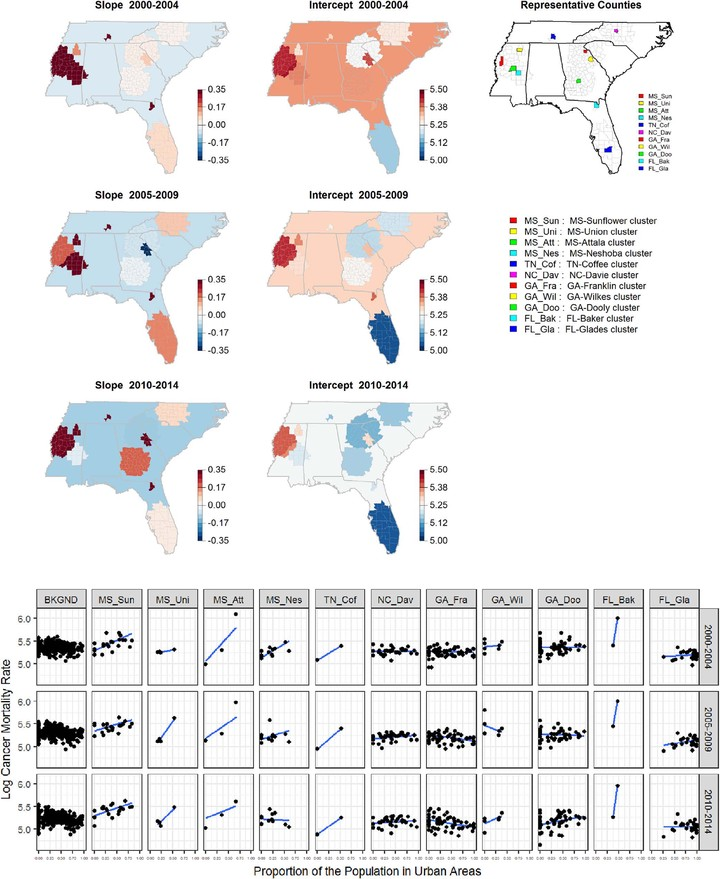# Clustered Spatio-Temporal Varying Coefficient Regression Model### Abstract

In regression analysis for spatio-temporal data, identifying clusters of spatial units over time in a regression coefficient could provide insight into the unique relationship between a response and covariates in certain subdomains of space and time windows relative to the background in other parts of the spatial domain and the time period of interest. In this article, we propose a varying coefficient regression method for spatial data repeatedly sampled over time, with heterogeneity in regression coefficients across both space and over time. In particular, we extend a varying coefficient regression model for spatial-only data to spatio-temporal data with flexible temporal patterns. We consider the detection of a potential cylindrical cluster of regression coefficients based on testing whether the regression coefficient is the same or not over the entire spatial domain for each time point. For multiple clusters, we develop a sequential identification approach. We assess the power and identification of known clusters via a simulation study. Our proposed methodology is illustrated by the analysis of a cancer mortality dataset in the Southeast of the U.S.

Type
Publication
Statistics in Medicine